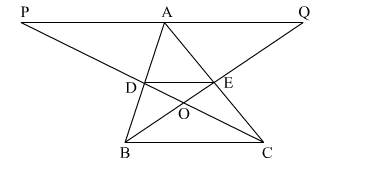# In the adjoining figure, D and E are respectively the midpoints of sides AB and AC of ∆ABC.

Question:

In the adjoining figure, D and are respectively the midpoints of sides AB and AC of ∆ABC. If PQ || BC and CDP and BEQ are straight lines then prove that ar(∆ABQ) = ar(∆ACP).Solution:

In $\triangle \mathrm{PAC}$

PA || DE and E is the midpoint of AC
So, D is the midpoint of PC by converse of midpoint theorem.

Also, $\mathrm{DE}=\frac{1}{2} \mathrm{PA}$     ........(i)

Similarly, $\mathrm{DE}=\frac{1}{2} \mathrm{AQ}$      ...........(ii)

From (1) and (2) we have
PA = AQ
∆ABQ and ∆ACP are on same base PQ and between same parallels PQ and BC
ar(∆ABQ) = ar(∆ACP)